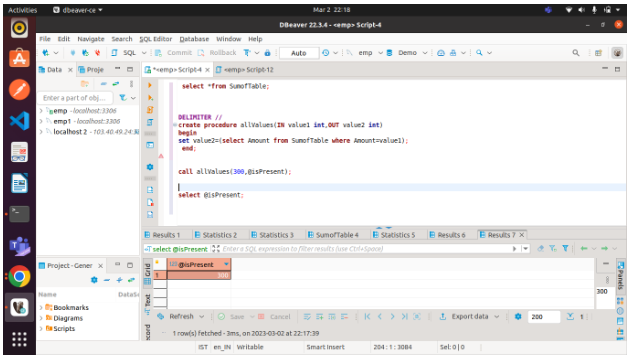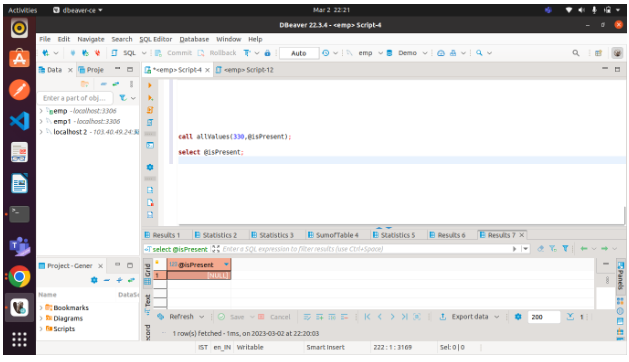/    /  MySQL – Stored Procedure Parameters

# MySQL Stored Procedure Parameters

The IN and OUT parameters can be used to create a parameter. Input parameters are taken from IN, and output parameters are taken from OUT.

syntax

```DELIMITER //
CREATE PROCEDURE yourProcedureName(IN yourParameterName dataType,OUT
yourParameterName dataType
)
BEGIN
Statement1;
Statement2;
.
.
N
END;
//
DELIMITER ;```

We will begin by creating a table. The query for creating a table is as follows:

```create table SumofTable
(Amount int );```

Using the insert command, add some records to the table.

Using the select statement, display all records from the table. Here is the query:

`select *from SumofTable;`In the next step, we will create a stored procedure that will determine whether the value is present in the table. You will receive a NULL value if the given value is not present in the table.

Here is the stored procedure:

```DELIMITER //
create procedure allValues(IN value1 int,OUT value2 int)
begin
set value2=(select Amount from SumofTable where Amount=value1);
end;```

In this example, we will call the stored procedure with some input and store the output in a session variable.

If the value is present:

`call allValues(300,@isPresent);`

Using the select statement, check the value of the variable @isPresent. Here is the query:

`select @isPresent;`The value is not present in the table:

`call allValues(330,@isPresent);`

Using the select statement, check the value of the variable @isPresent. Here is the query:

`select @isPresent;`